How Cheenta works to ensure student success?
Explore the Back-Story

# Problem related to triangle - AMC 10B, 2019 Problem 10The given problem is related to the calculation of area of triangle and distance between two points.

## Try the problem

In a given plane, points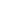and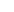are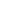units apart. How many pointsare there in the plane such that the perimeter of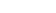is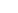units and the area ofis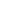square units?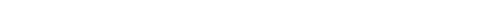2019 AMC 10B Problem 10

Problem related to triangle

6 out of 10

Secrets in Inequalities.

## Use some hints

Notice that it does not matter where the triangle is in the 2D plane so for our easy access we can select two points A and B in any place of choice.

So we can actually select any two points A and B such that they are 10 units apart so lets the points are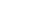and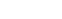, as they are 10 units apart.

Now we can select the point C such that the perimeter of the triangle is 50 units. and then we can apply the formula of area to calculate the possible positions of C.

## Subscribe to Cheenta at Youtube

The given problem is related to the calculation of area of triangle and distance between two points.

## Try the problem

In a given plane, pointsandareunits apart. How many pointsare there in the plane such that the perimeter ofisunits and the area ofissquare units?2019 AMC 10B Problem 10

Problem related to triangle

6 out of 10

Secrets in Inequalities.

## Use some hints

Notice that it does not matter where the triangle is in the 2D plane so for our easy access we can select two points A and B in any place of choice.

So we can actually select any two points A and B such that they are 10 units apart so lets the points areand, as they are 10 units apart.

Now we can select the point C such that the perimeter of the triangle is 50 units. and then we can apply the formula of area to calculate the possible positions of C.

## Subscribe to Cheenta at Youtube

This site uses Akismet to reduce spam. Learn how your comment data is processed.

### Knowledge Partner